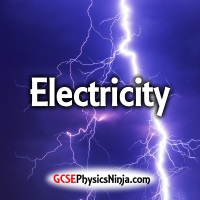# ElectricityWelcome to the GCSE Physics Ninja Electricity course.

These video lessons are based on question-and-answer flashcards. You can either make your own or save time by purchasing the printable flashcard set from the shop.

## What will I learn in this course?

When you have completed this course, you will be able to...

• Describe and understand static electricity.
• Explain how to produce and detect electrical charges.
• Understand the nature of electric fields.
• Describe electric current as a flow of charge and perform calculations.
• Define potential difference in terms of energy and charge.
• Calculate the electrical work done by charge as it flows through a component.
• Recognise current vs. potential difference graphs for various components.
• Use the current, resistance & potential difference equation in calculations.
• Describe how the resistance of a filament bulb varies with current.
• Explain how resistance of a wire varies with length and diameter.
• Analyse series and parallel circuits.
• Find the total resistance of a series or parallel network of resistors.
• Describe and recognise common electrical components.
• Understand how potential dividers work.
• Appreciate the differences between analogue and digital signals.
• Analyse and design digital circuits using logic gates.
• Describe the characteristics of the mains electricity supply.
• Explain household electricity safety precautions.
• Calculate the electrical energy transferred using the power rating of an appliance.
• Understand that electrical energy can be measured in joules or kilowatt-hours.